# Mr. Meinzen - Advanced Placement Calculus AB

### Cooperative Learning Assessment Bonus Score Sheet

 Assessment -- Test or Quiz Info (example: Quiz 1.1-1..3 or Test Chapter 1):

Calculus Peer Names & Roles
Quiz or Test Percentage

Average Team Percentage
(calculated by team----round DOWN)
 Team or Class Average (%) BonusPercentage Points 90 3 85 2.5 80 2 75 1.5 70 1
Average Class Percentage
(provided by teacher):

Team Bonus
(see table on left):

Class Bonus
(see table on left):

TOTAL BONUS PERCENTAGE POINTS
(add team bonus + class bonus then -round UP)

Instructions:

Teacher Prints Out Sheets

then Students:

1. Cut Out Each Graph,
2. Match Pairs of Function Graphs with
3. corresponding Derivative Graphs
 Letter of Function Graph Matching Letter of Derivative GraphA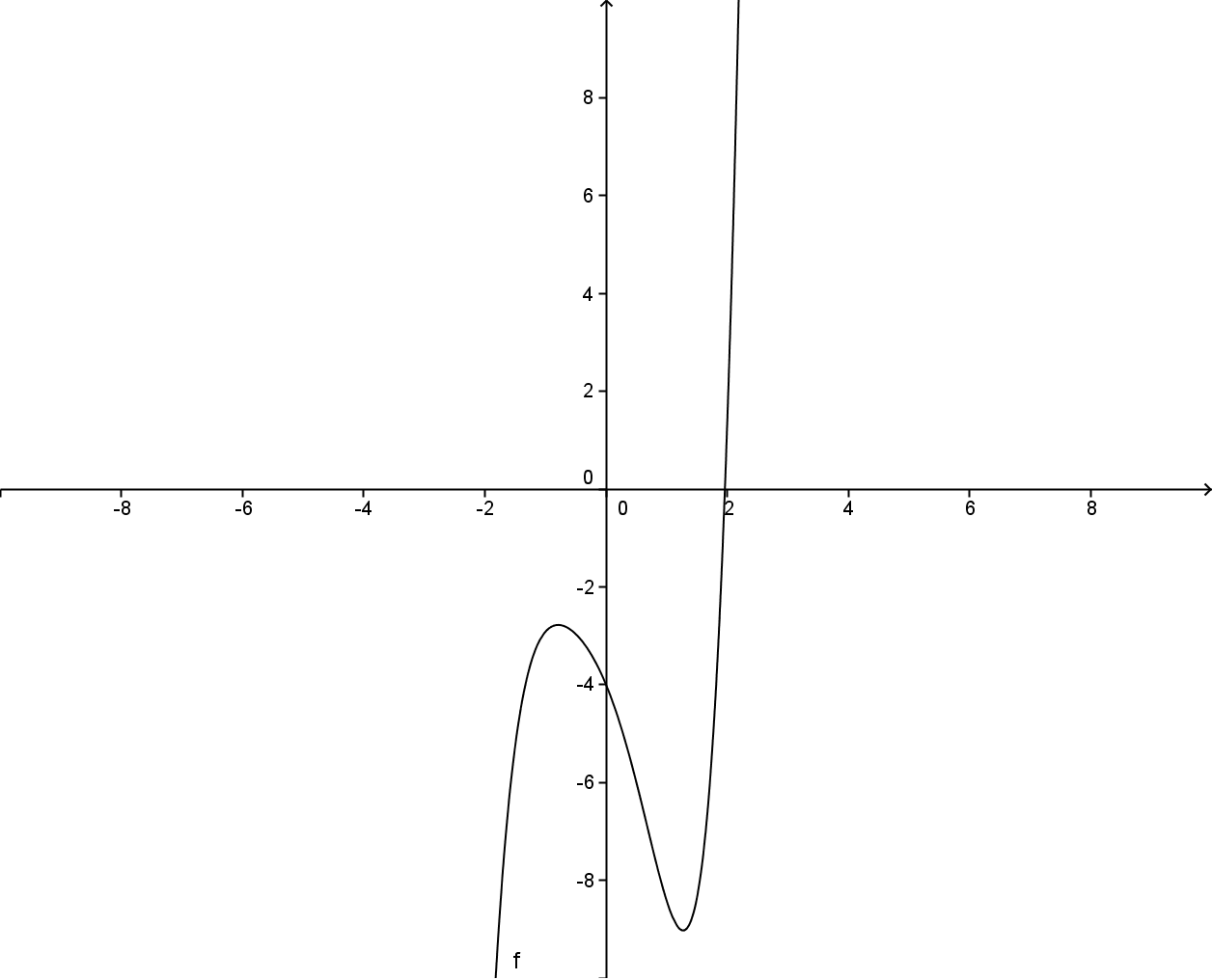BTC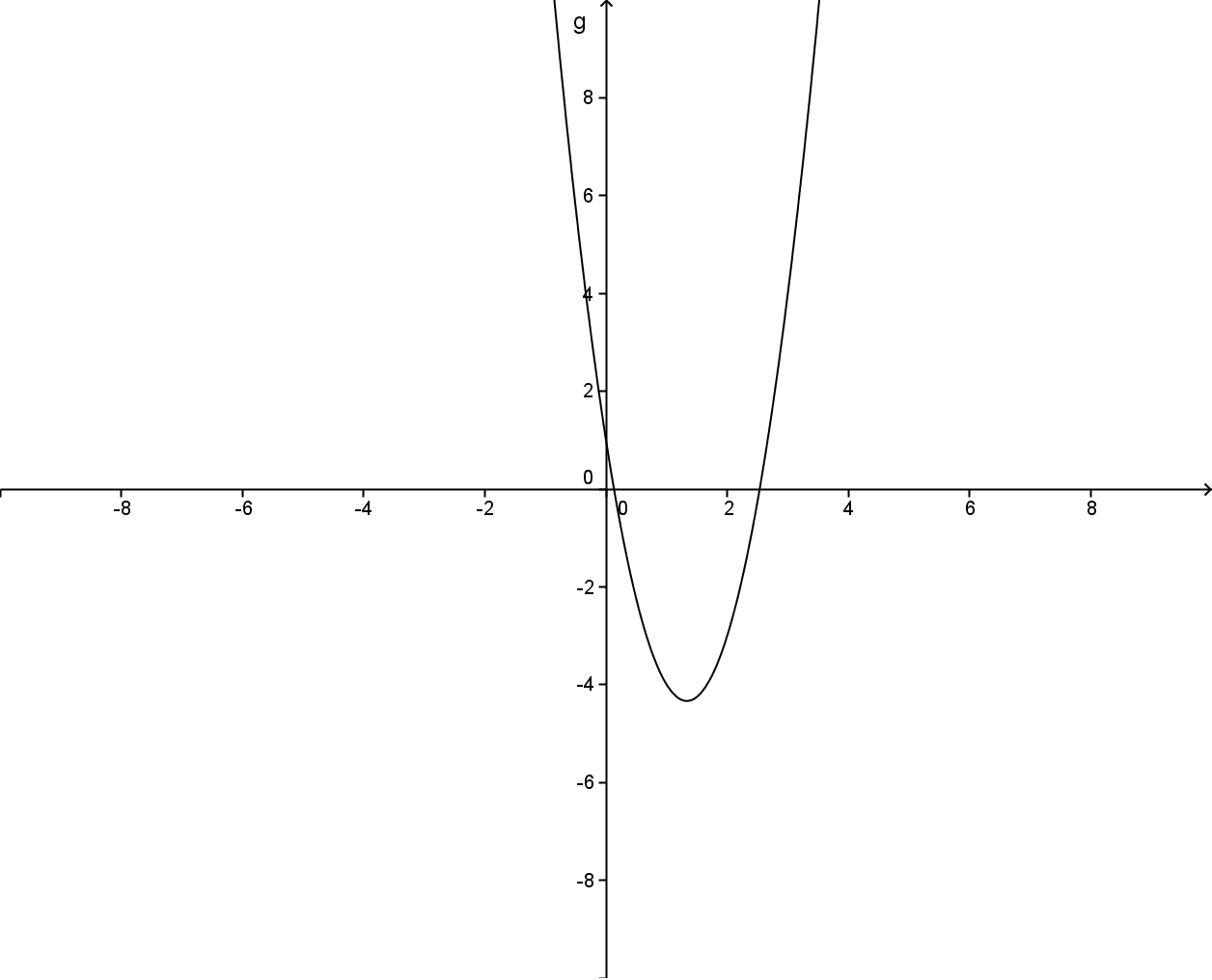XQ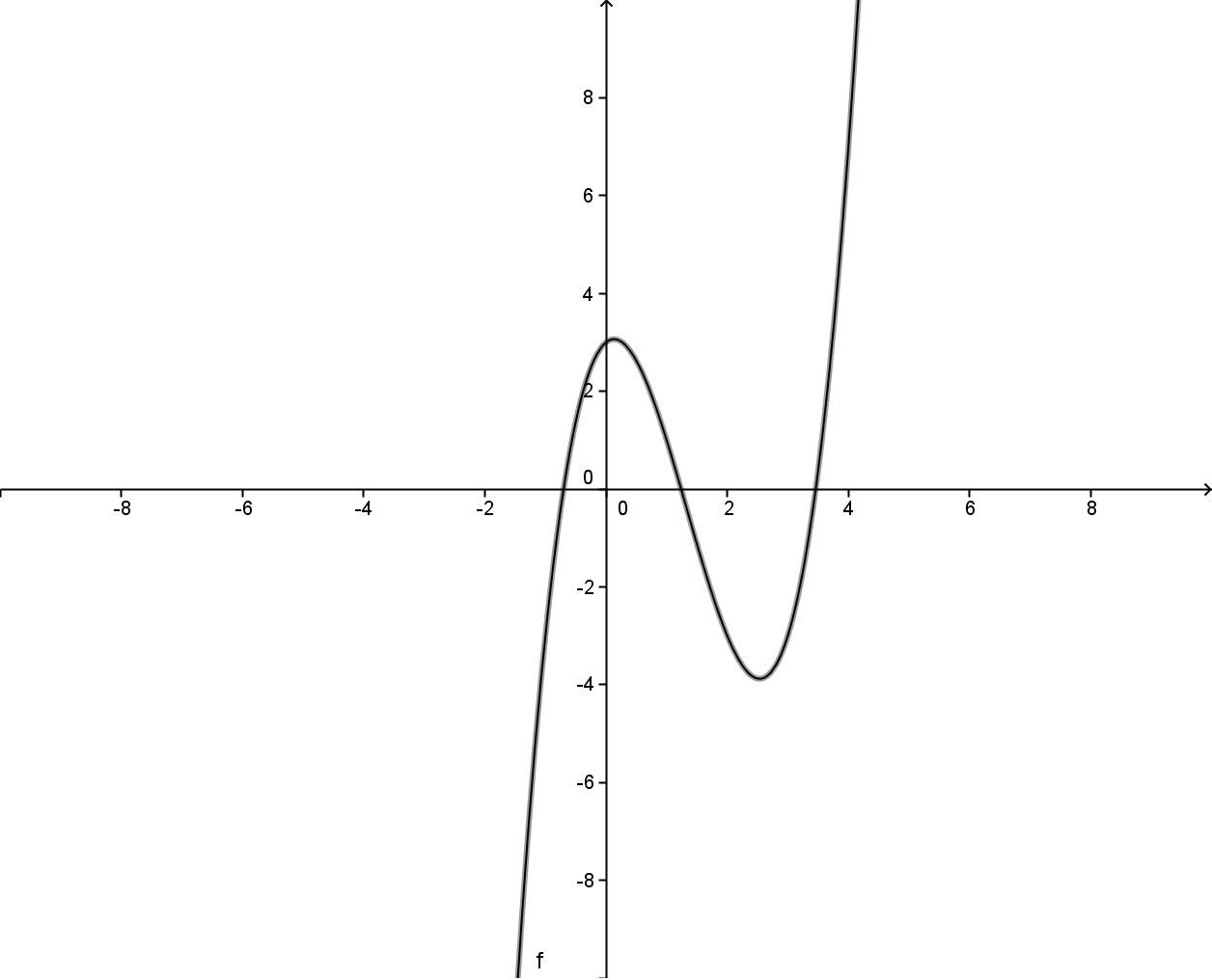D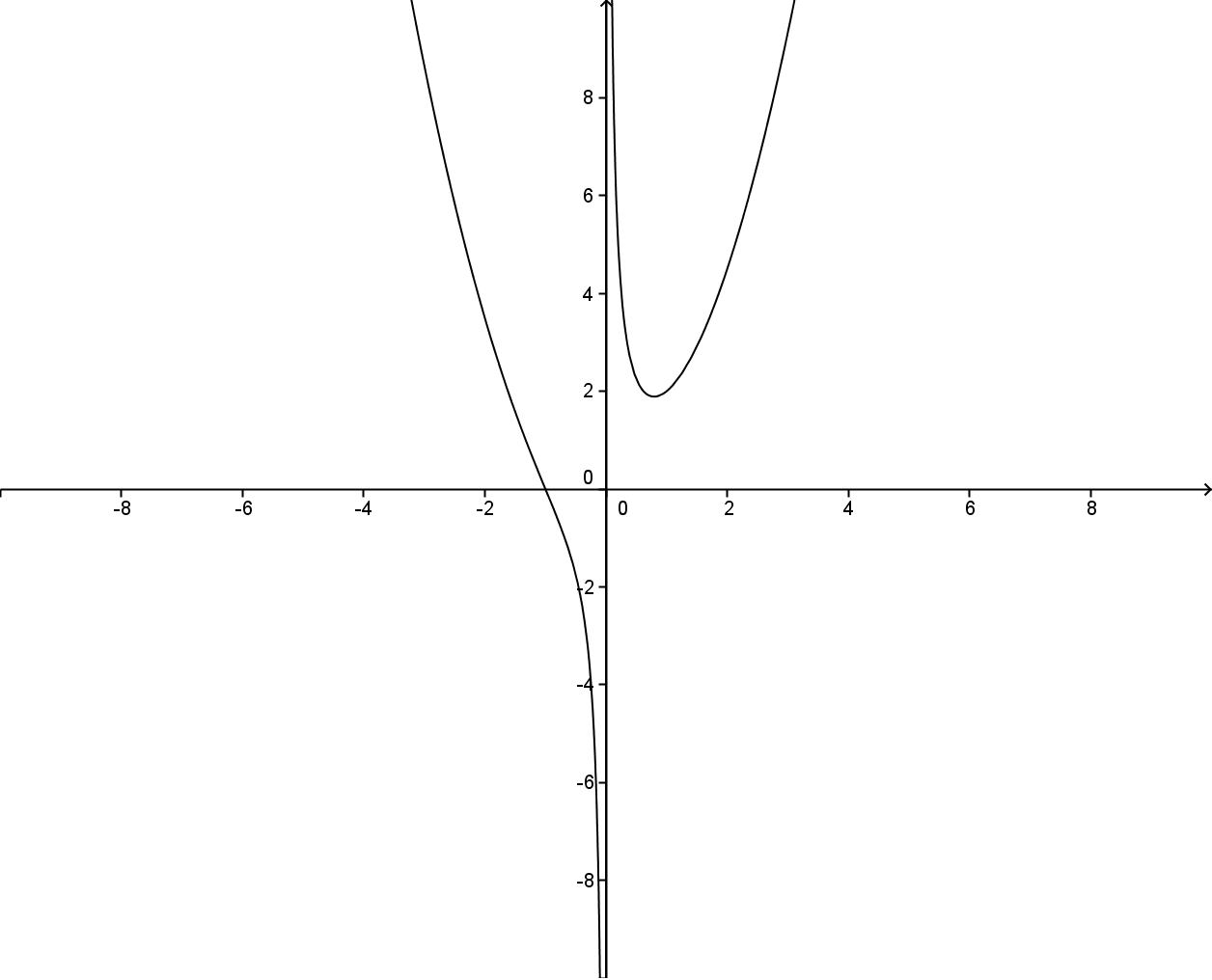EVBBF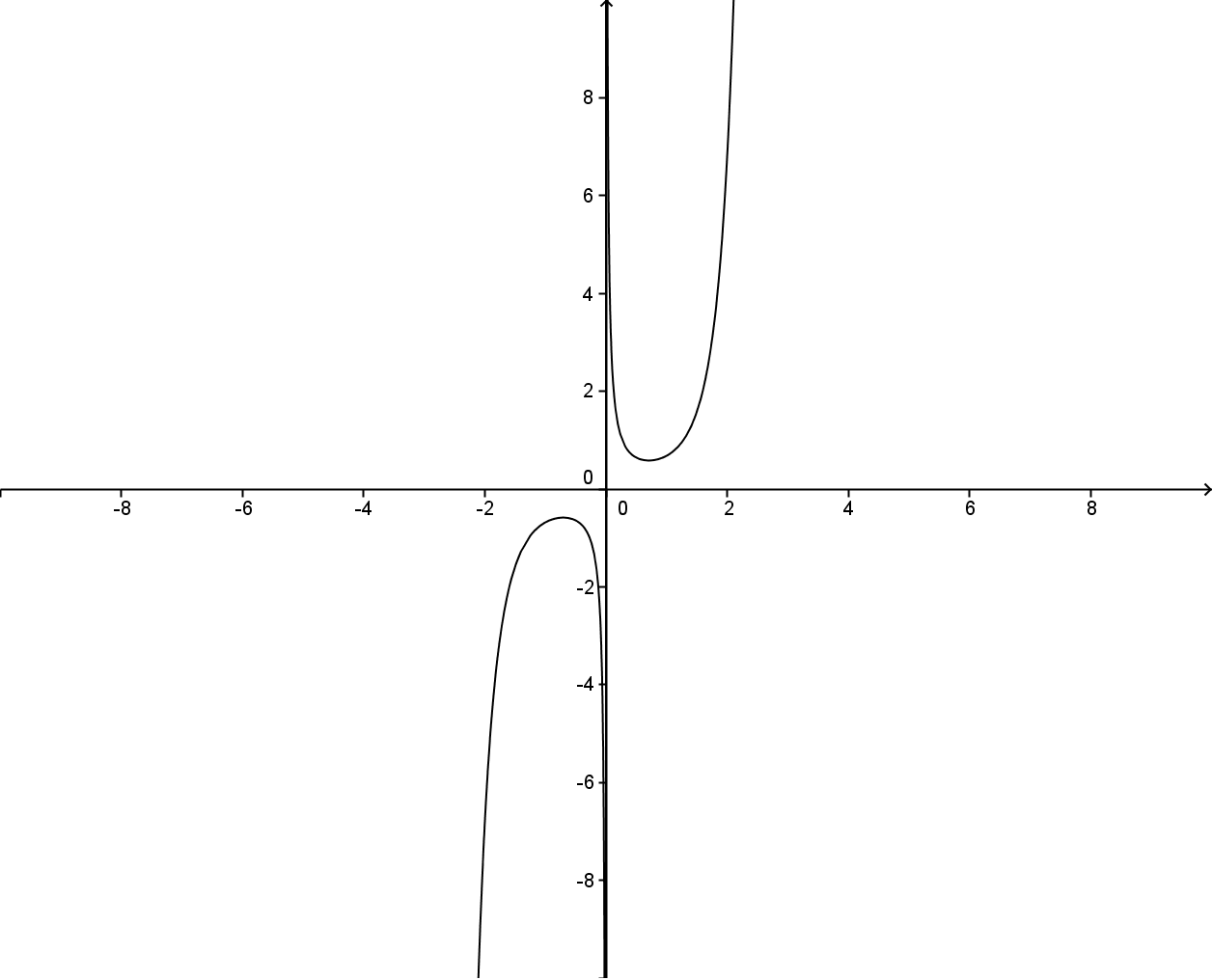GS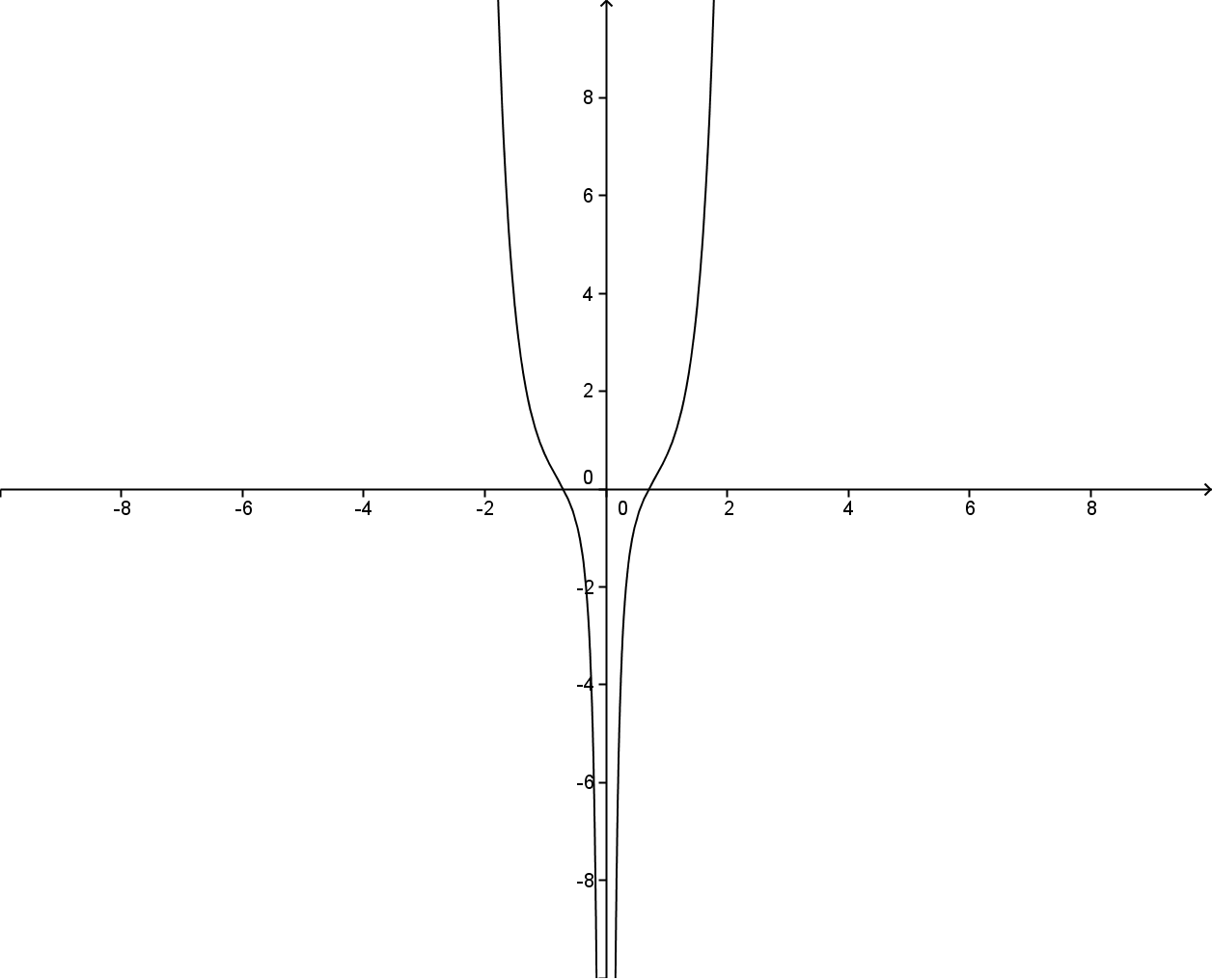Y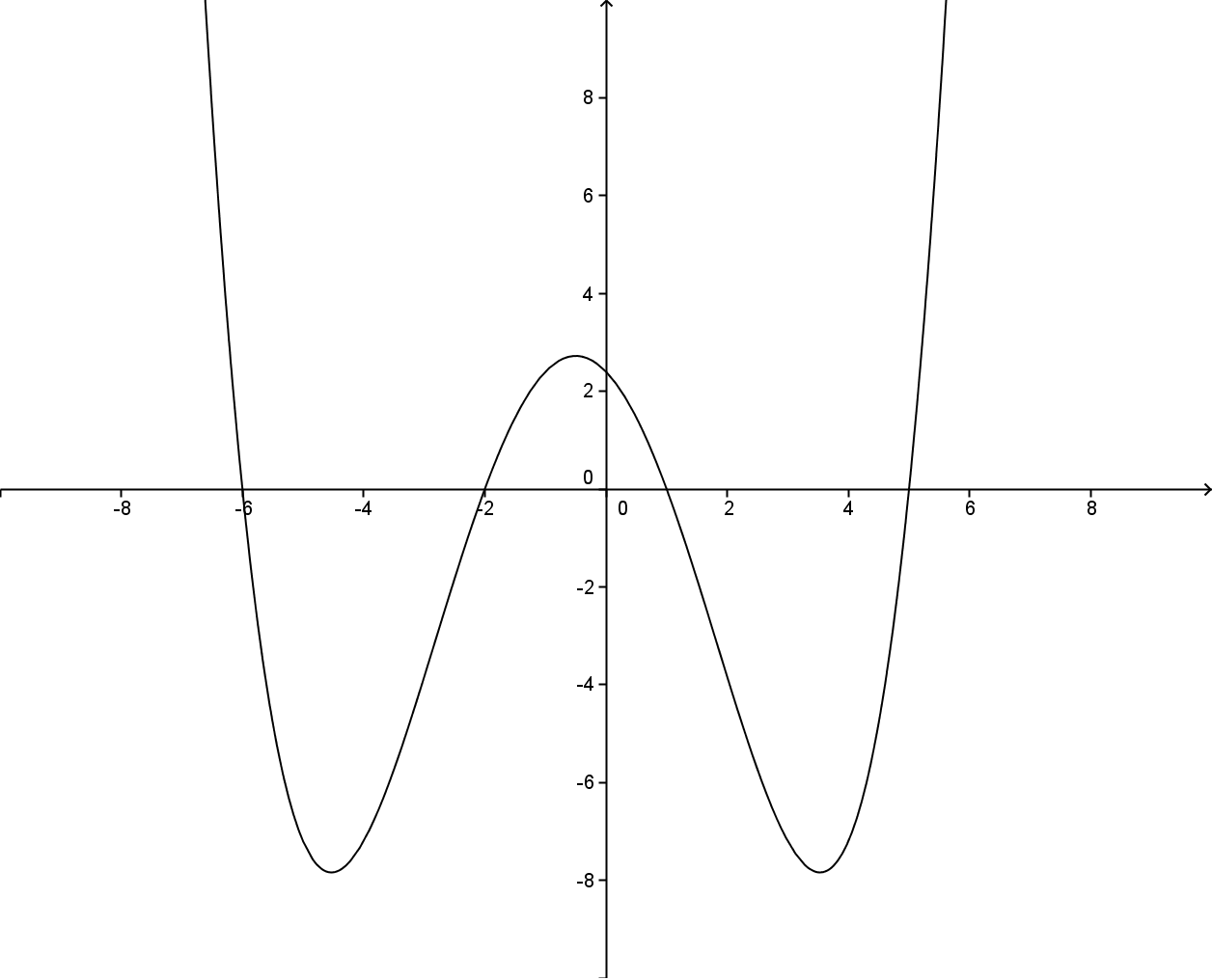H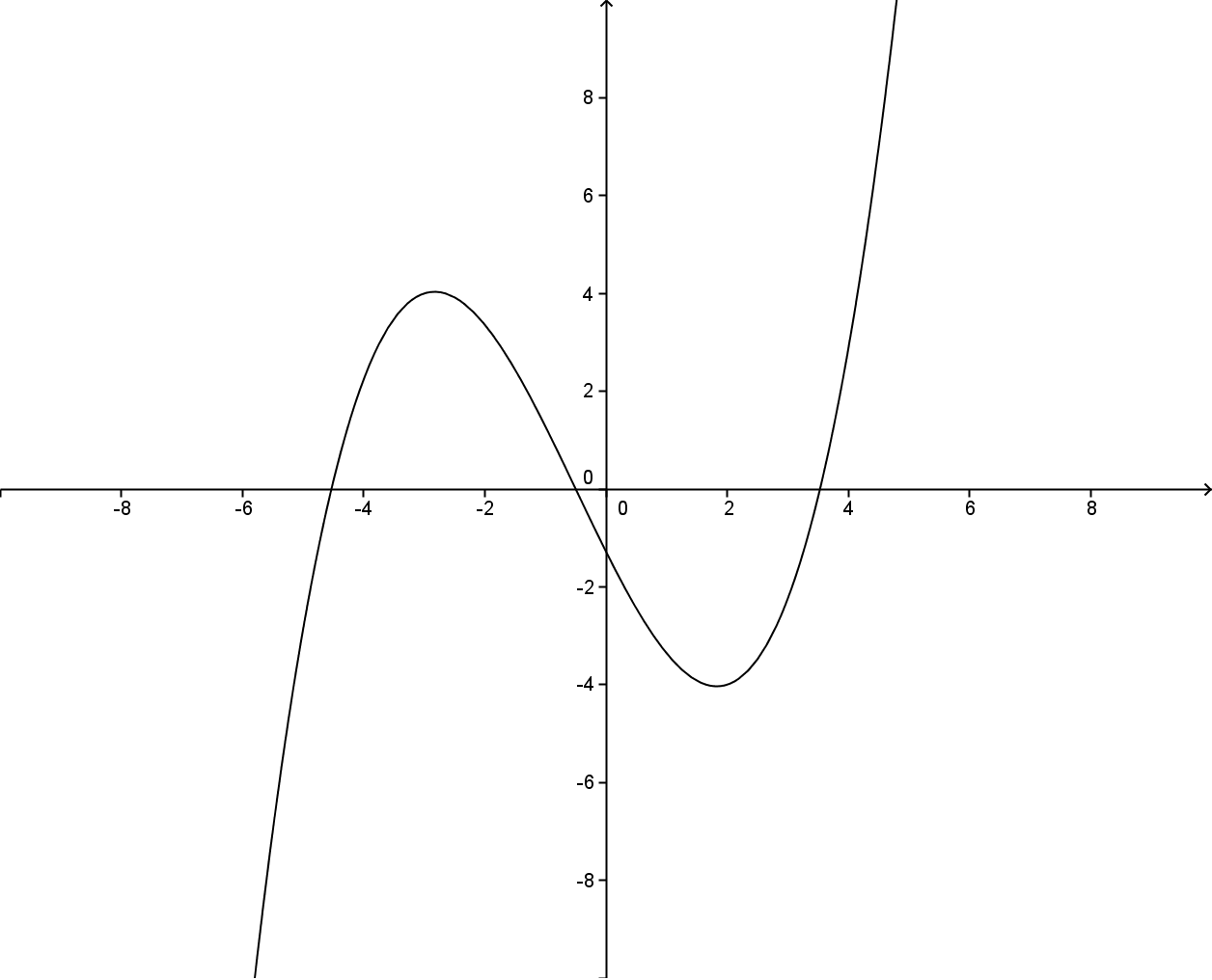FF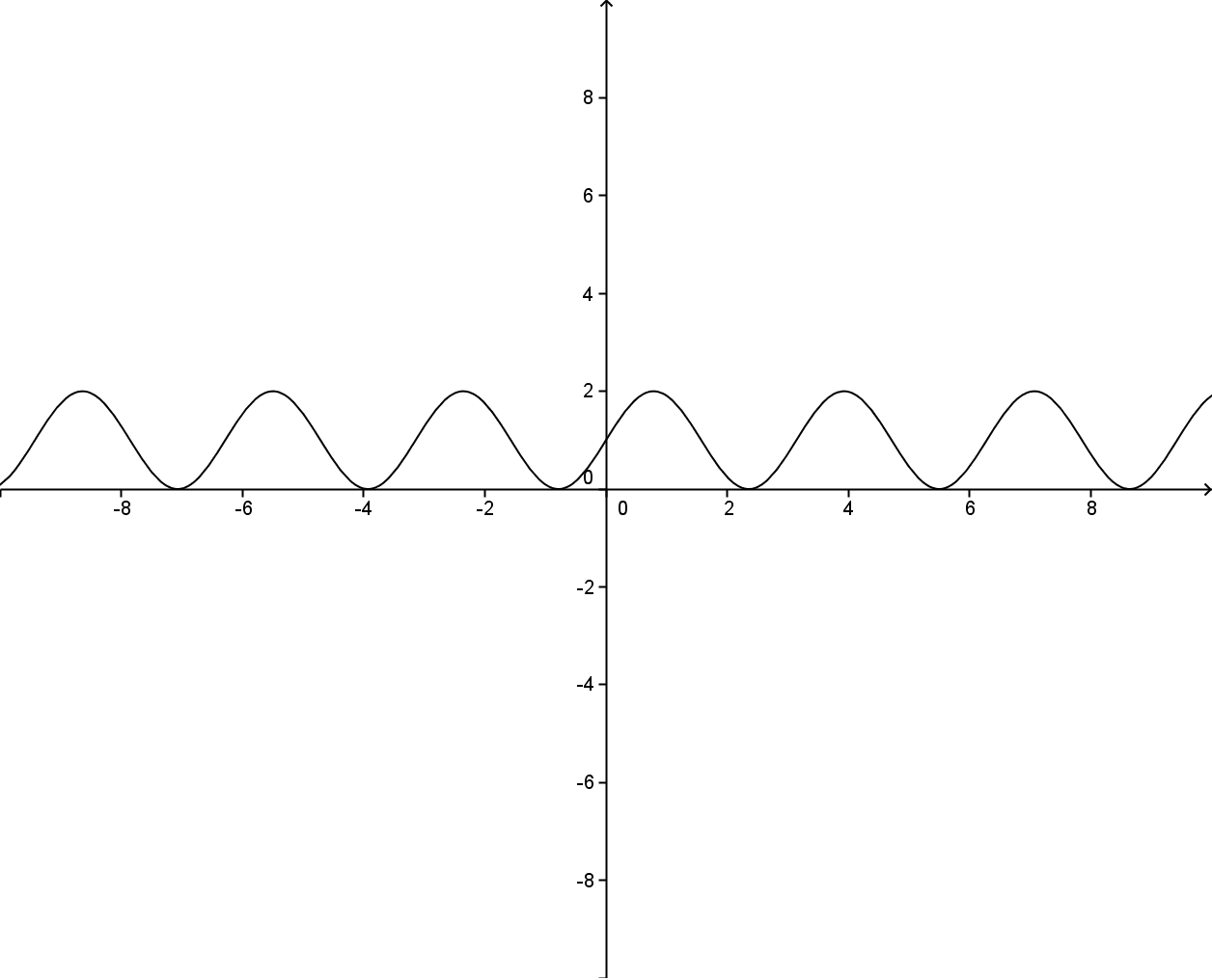WK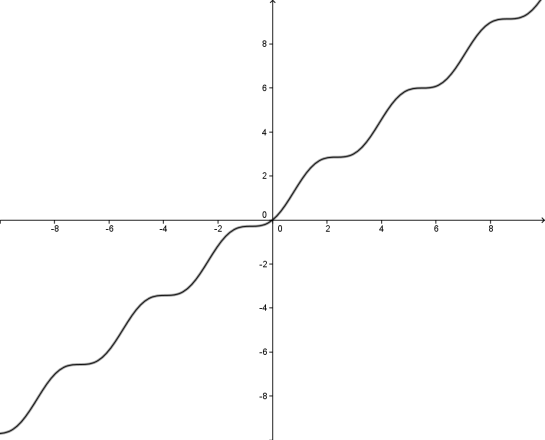I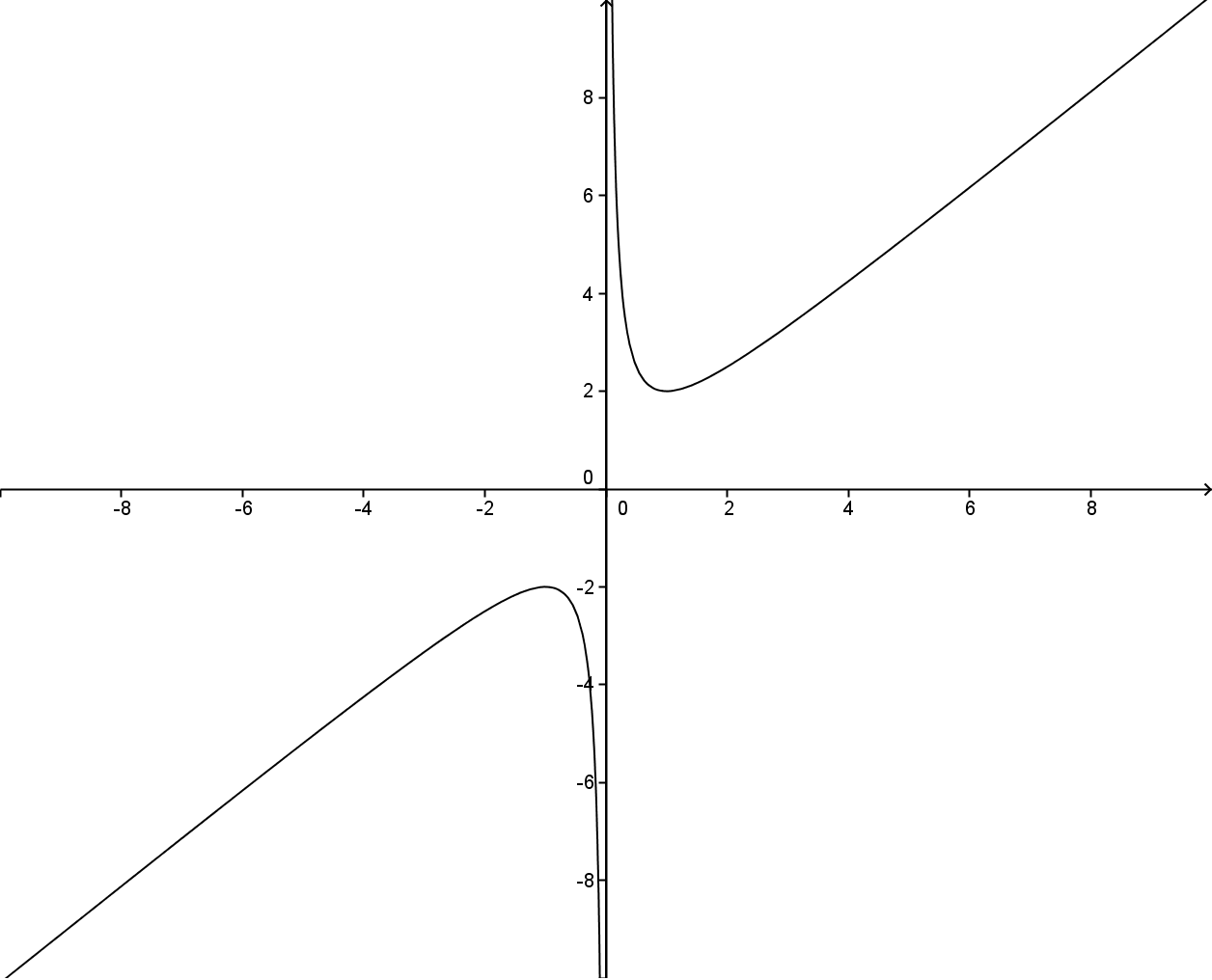JAAL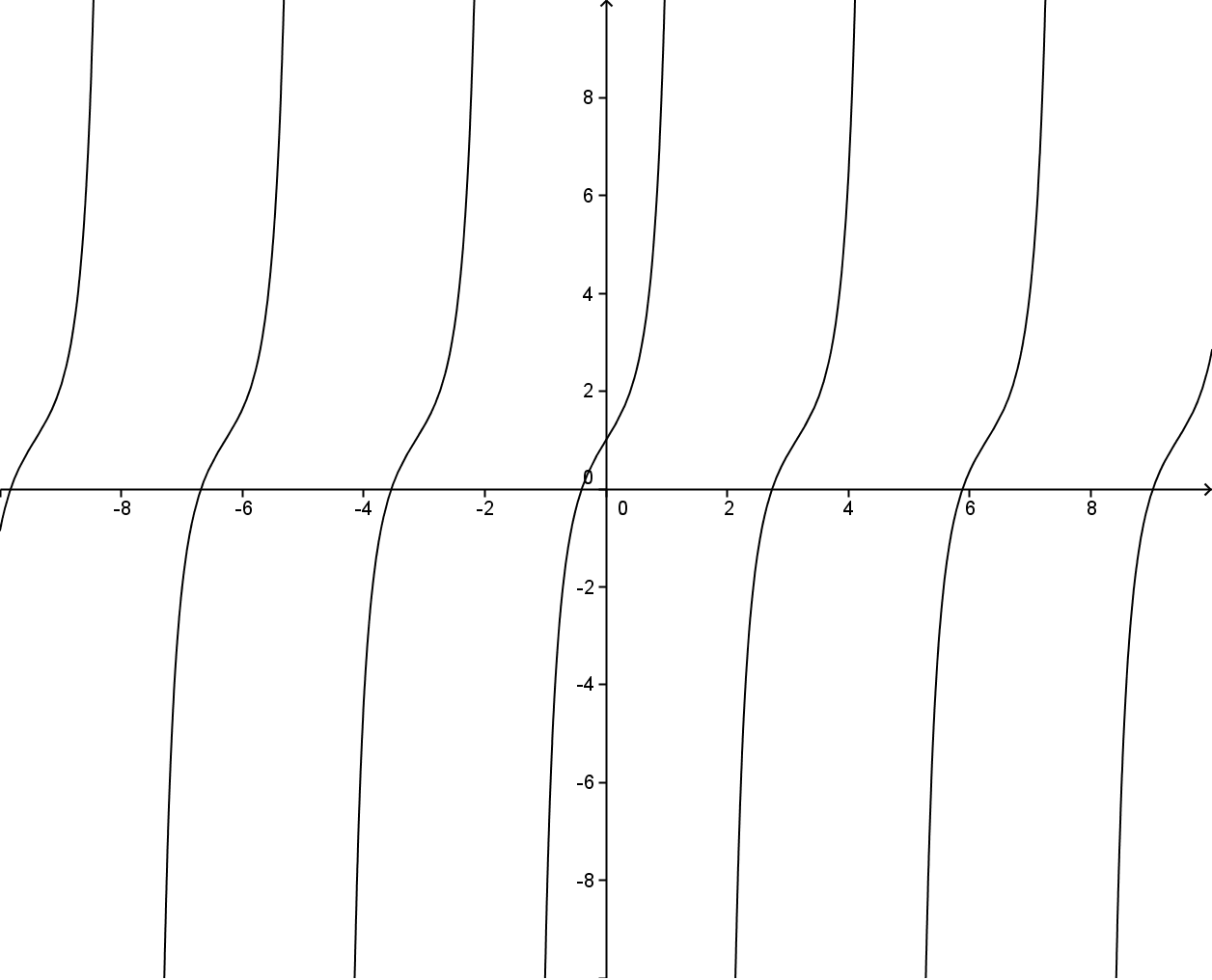CC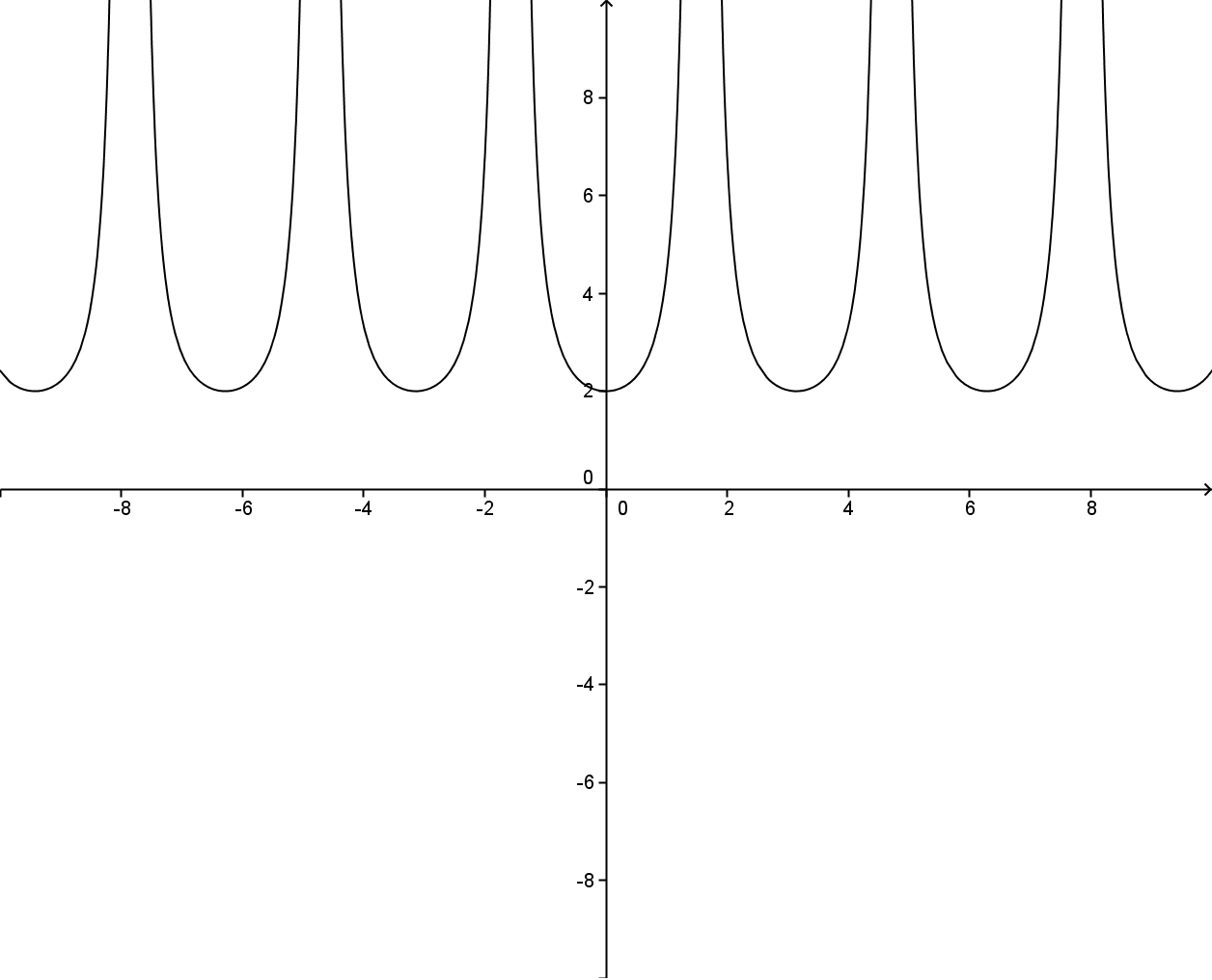R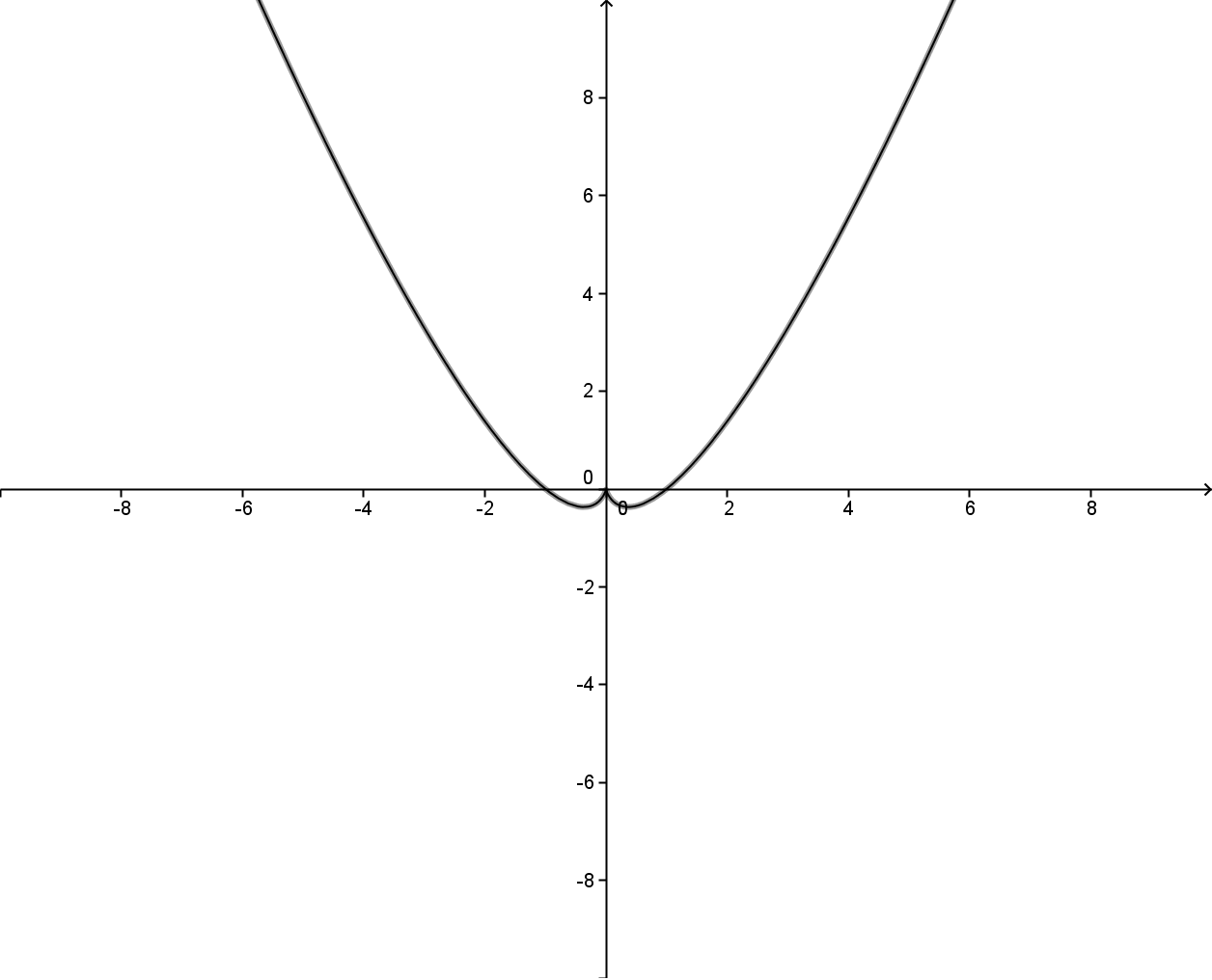MN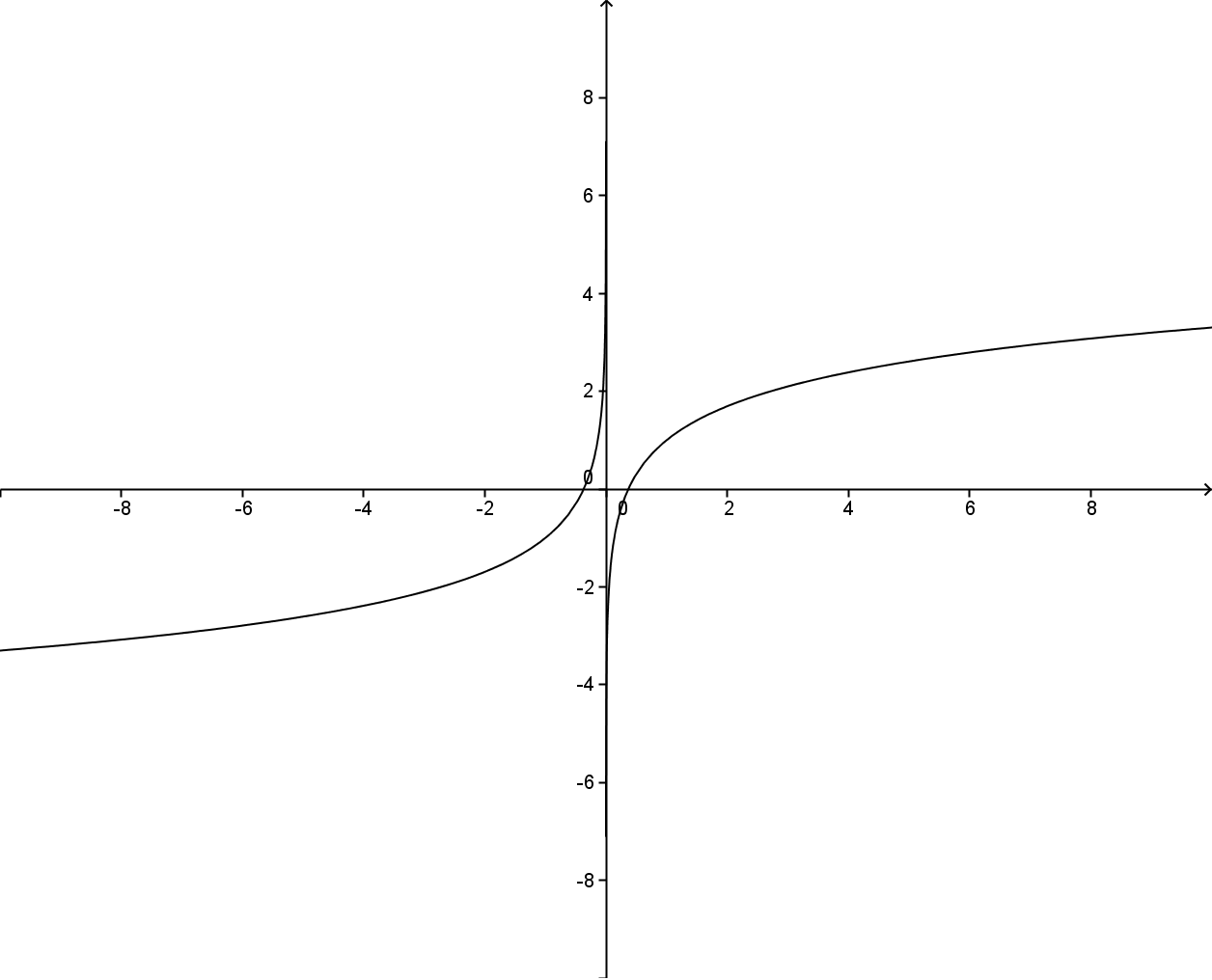EE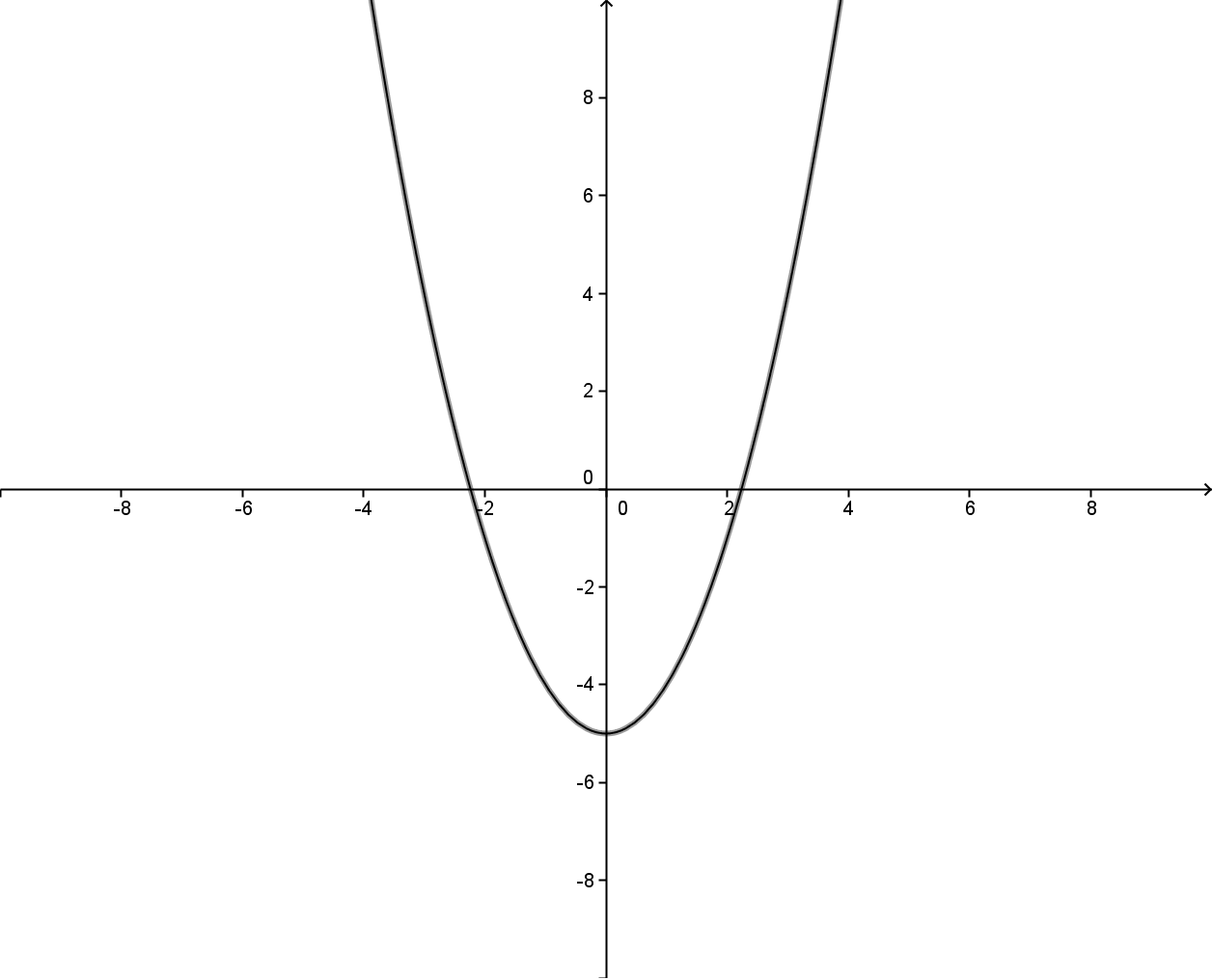OUZPDD
 Letter of Function Graph Matching Letter of Derivative Graph A T B Q C V D X E BB F S G Y H FF I W J AA K R L CC M EE N Z O U P DD

### Select AP Exam Questions

#### From 2010 AP Calculus BC Free Response #5.

Let f and g be the functions defined by f(x) = 1/x and g(x) = (4x) / (1+4x^2) for all x > 0.

(a)

Find the absolute maximum value of g on the open interval (0, infinity) if the maximum exists.

Find the absolute minimum value of g on the open interval (0, infinity) if the minimum exists.